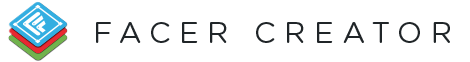#Conditionals are the most complicated and dynamic way to create a watch face.

They take this form:

``` \$ EQUALITY TEST ? EXPRESSION IF TEST IS TRUE : EXPRESSION IF TEST IS FALSE \$```

The first space is for our equality test, which is going to equal true or false. There are five types of operators available:

OPERATOR DEFINITION EXAMPLE EXPRESSION EXAMPLE OUTPUT
= or == Is equal to (1=1) or (1==1) true
!= Is not equal to (1!=0) true
> Is greater than (2>1) true
< Is less than (0<1) true
>= Is greater than or equal to (2>=1) true
<= Is less than or equal to (0<=1) true

Now let's make our own. We'll fill it in step-by-step. Let's start with a blank slate. We'll develop a conditional to use for the Transparency attribute. All of the those dollar signs and question marks look confusing at first, but you'll get used to them (if you have a programming background, you probably already recognize the ternary operator!):

`\$____________?_:_\$`

Now let's fill in that spot with a simple example. We're going to make an expression that will make a layer appear only before noon. This expression would be added to the opacity attribute of the layer. We'll use the #Da# and check if it's "AM":

``` \$#Da#==AM?_:_\$```

Next, we'll fill in the EXPRESSION IF TRUE part of the conditional. When #Da# == "AM" is true, we want our layer to appear, so we'll want the opacity to be 100:

`\$#Da#==AM?100:_\$`

Lastly, we'll fill in the EXPRESSION IF FALSE part of the conditional. When #Da# == "AM" is false, we want our layer to disppear, so we'll want the opacity to be 0:

``` \$#Da#==AM?100:0\$```

That's it! You just created your first conditional.

Don't use spaces (" ") before and after part of conditions (wrong: `\$ #Da# == AM ? 100 : 0 \$` right: `\$#Da#==AM?100:0\$`)